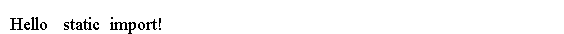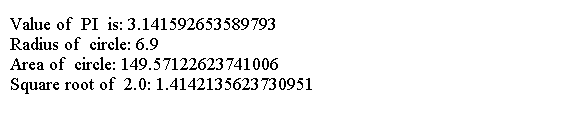# Java 导入

• 单类型导入声明
• 按需导入声明

## 单类型导入声明

```import <fully qualified name of a type>;
```

```import com.w3cschool.Dog;
```

```import pkg1.ClassOne;
import pkg2.ClassTwo;
import pkg2.ClassThree;
import pkg3.ClassFour;
```

```public  class  Main{
public static  void  main(String[]  args)  {
com.w3cschool.Dog jack;  // Uses  full qualified name for the   Dog  class
}
}
```

```import com.w3cschool.Dog; // Import   the   Dog  class

public  class  Main {
public static  void  main(String[]  args)  {
Dog  jack; // Use simple name of  the   Dog  class
}
}
```

```Dog  jack;
```

```com.w3cschool.Dog jack;
```

## 按需导入声明

```import <package name>.*;
```

```import com.w3cschool.*;
```

```import com.w3cschool.*;

public  class  Main {
public static  void  main(String[]  args)  {
Dog  jack; // Use simple name of  the   Dog  class
}
}
```

## 静态导入声明

```import static <package  name>.<type name>.<static member name>;
```

```import static <package  name>>.<type  name>.*;
```

```import static  java.lang.System.out;
```

```import static  java.lang.System.out;

public class  StaticImportTest  {
public static  void  main(String[]  args)  {
out.println("Hello static  import!");
}
}
```java.lang包中的Math类有许多实用程序常量和静态方法。

```import static  java.lang.Math.*;
```

## 例子

```import static java.lang.Math.PI;
import static java.lang.Math.sqrt;
import static java.lang.System.out;

public class Main {
public static void main(String[] args) {

out.println("Value of  PI  is: " + PI);
out.println("Area of  circle: " + area);
out.println("Square root of  2.0: " + sqrt(2.0));
}
}
```• 如果导入具有相同简单名称的两个静态成员，一个使用单静态导入声明，另一个使用静态import-on-demand声明，则使用单静态import声明导入的静态成员优先。
• 使用单静态导入声明来导入具有相同简单名称的两个静态成员是不允许的。
• 如果使用单静态导入声明导入静态成员，并且在同一类中存在具有相同名称的静态成员，则使用类中的静态成员。

App下载